# Difference between revisions of "Strong law of large numbers"

A form of the law of large numbers (in its general form) which states that, under certain conditions, the arithmetical averages of a sequence of random variables tend to certain constant values with probability one. More exactly, let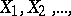(1)

be a sequence of random variables and let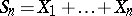. One says that the sequence (1) satisfies the strong law of large numbers if there exists a sequence of constants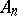such that the probability of the relationship(2)

is one. Another formulation, which is equivalent to the former one, is as follows: The sequence (1) satisfies the strong law of large numbers if, for any, the probability of all the inequalities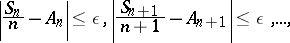(3)

to be true at the same time tends to one as. Thus, one considers the behaviour of the sequence of the sums as a whole, whereas in the ordinary law of large numbers individual sums only are considered. If the sequence (1) satisfies the strong law of large numbers, it also satisfies the ordinary law of large numbers for the same, i.e.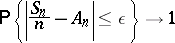(4)

for anyif. The converse need not be true. For example, if the random variables (1) are independent and, for, assume the two values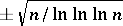with probability 1/2 each, they satisfy the law of large numbers (4) for, but the strong law of large numbers (2) is not satisfied for any value of. The existence of such examples is not at all obvious at first sight. The reason is that even though, in general, convergence in probability is weaker than convergence with probability one, nevertheless the two types of convergence are equivalent, for example, in the case of series of independent random variables.

The strong law of large numbers was first formulated and demonstrated by E. Borel  for the Bernoulli scheme in the number-theoretic interpretation; cf. Borel strong law of large numbers. Special cases of the Bernoulli scheme result from the expansion of a real number, taken at random (with uniform distribution) in the interval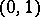, into an infinite fraction to any basis (see Bernoulli trials). Thus, in the binary expansion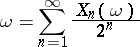the successive signs ofassume two values, 0 and 1, with probability 1/2 each, and are independent random variables. The sum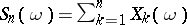is equal to the number of ones among the firstsigns in the binary expansion, whileis their proportion. At the same timemay be considered as the number of "successful" trials in the Bernoulli scheme with probability of "success" (appearance of "1" ) equal to 1/2. Borel showed that the proportion of "ones" ,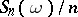, tends to 1/2 for almost-allin. In a similar manner, in the expansion ofto the base 10, "success" may be considered as the appearance of any one of the digits(e.g. the number 3). One then obtains a Bernoulli scheme with probability of success 1/10 and the frequency of appearance of the selected digit among the firstsigns in the decimal expansion tends to 1/10 for almost-allin. It was also noted by Borel that the frequency of appearance of any given group ofdigits tends to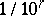for almost-all(cf. Normal number).

F. Cantelli  stated sufficient conditions for the strong law of large numbers for independent random variables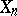in terms of the second and fourth central moments of the summands (these conditions are fulfilled for the Bernoulli scheme). Putting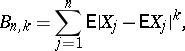the Cantelli condition can be written in the form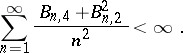The proofs of Cantelli and Borel are based on the following reasoning. Let, for some sequence of positive numbers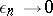(when),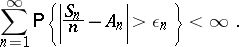(5)

Then, according to the Borel–Cantelli lemma, only a finite number of events under the probability sign in (5) are realized with probability one. Accordingly, for all sufficiently large,with probability one, i.e. (3) is valid. Borel estimated the terms of the series (5) by the de Moivre–Laplace theorem, while Cantelli did so basing himself on Chebyshev's inequality with fourth moments.

A further extension of the conditions for the applicability of the strong law of large numbers was realized by A.Ya. Khinchin and A.N. Kolmogorov. Khinchin ,  introduced the very name of "strong law of large numbers" and proved a sufficient condition (also applicable to dependent variables) for it with. Denoting by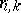the correlation coefficient between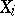and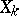and putting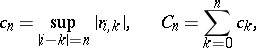one can write Khinchin's condition as follows: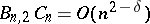for some. In fact, the statement which follows from Khinchin's proof is much stronger.

In the case of independent summands the best known conditions for applicability of the strong law of large numbers are those established by Kolmogorov: Sufficient (1930) for variables with a finite variance and necessary and sufficient conditions (1933) for identically-distributed variables consist of the existence of the mathematical expectation of the variables. Kolmogorov's theorem for random variables (1) with finite variances states that the condition(6)

implies the applicability of the strong law of large numbers with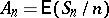for the sequence (1). In terms of variances condition (6) is best in the sense that for any sequence of positive numbers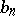such that the seriesdiverges it is possible to construct a sequence of independent random variableswith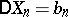which does not satisfy the strong law of large numbers. The scope of application of condition (6) (and also of other conditions of the strong law of large numbers for independent variables) may be extended as follows. Letbe the median of. The convergence of the series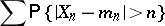is necessary in the strong law of large numbers. It follows from the Borel–Cantelli lemma that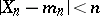with probability one, starting from a certain number. Accordingly, in a study on the conditions of applicability of the strong law of large numbers, one may immediately restrict to random variables which satisfy the last-named condition.

In the proof given by Khinchin and Kolmogorov the convergence of the series (5) is replaced by the convergence of the series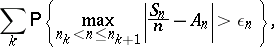where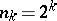. In this proof, Khinchin actually employed a number of ideas from the theory of orthogonal series of functions, while Kolmogorov employed his inequality for the maxima of sums of random variables.

It is possible to state a necessary and sufficient condition for the strong law of large numbers for independent random variables. Putting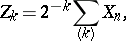where the sumapplies to the values offor which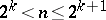, this condition may be written as follows: For any,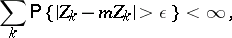(7)

where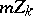is the median of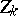. If additional restrictions are imposed, (7) will yield conditions expressed in terms of the characteristics of the individual terms. For instance, if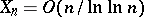or if allare normally distributed, condition (7) is equivalent to the following one: For any,(8)

Here, sinceare independent,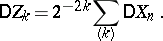Conditions of applicability of the strong law of large numbers to Markov chains and processes, and to stationary processes, are known . Thus, Khinchin's method, which is applicable to a sequencethat is stationary in the wide sense, with correlation function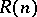, leads to the following theorem: If the series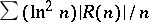is convergent, thenwith probability one. In applications to stationary (in the narrow sense) random processes the name "strong law of large numbers" is sometimes given to the following statement: The limit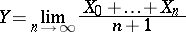exists with probability one. The random variableis equal to the conditional mathematical expectation of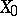with respect to the-algebra of sets that are shift-invariant; the variableis constant and equalswith probability one only for metrically-transitive processes. The strong law of large numbers in this form is identical with the Birkhoff ergodic theorem.

There exist variations of the strong law of large numbers for random vectors in normed linear spaces . The chronologically earliest example of such a variation is the Glivenko–Cantelli theorem on the convergence of the empirical distribution function to the theoretical one.

The deviations of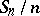fromare described by the law of the iterated logarithm.

How to Cite This Entry:
Strong law of large numbers. Encyclopedia of Mathematics. URL: http://encyclopediaofmath.org/index.php?title=Strong_law_of_large_numbers&oldid=17949
This article was adapted from an original article by Yu.V. Prokhorov (originator), which appeared in Encyclopedia of Mathematics - ISBN 1402006098. See original article